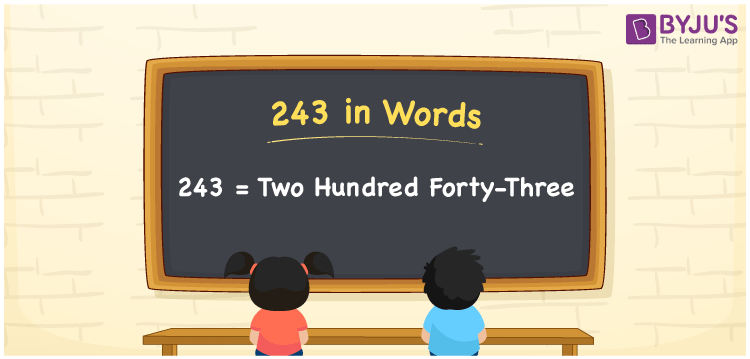# 243 in Words

We can write 243 in words as Two hundred forty-three. If you purchased an ointment that costs Rs. 243, then you can say, “I purchased an ointment worth Two hundred forty-three rupees”. Let’s learn how to convert the cardinal number 243 into words using a place value chart and some facts about 243 here in this article.

 243 in words Two hundred forty-three Two hundred forty-three in Numbers 243

## 243 in English Words

We generally write numbers in words using the English alphabet. So, we can spell 243 in English words as “Two hundred forty-three”.## How to Write 243 in Words?

As we can see, 243 is a three-digit number so, let’s create a three-column place value chart, as given below.

 Hundreds Tens Ones 2 4 3

Here, ones = 3, tens = 4, hundreds = 2

Let us expand these digits according to their place values.

2 × Hundred + 4 × Ten + 3 × One

= Two hundred + Forty + Three

= Two hundred forty-three

Hence, 243 in words = Two hundred forty-three

243 is a natural number that precedes 244 and succeeds 242.

243 in words – Two hundred forty-three

Is 243 an odd number? – Yes

Is 243 an even number? – No

Is 243 a prime number? – No

Is 243 a composite number? – Yes

Is 243 a perfect square number? – No

Is 243 a perfect cube number? – No

## Frequently Asked Questions on 243 in Words

Q1

### What is 243 in word form?

Two hundred forty-three represents the number 243 in word form.
Q2

### What is the spelling of 243?

The spelling of 243 in English words is “Two hundred forty-three”.
Q3

### Express 243.56 in words.

We can express the decimal number 243.56 in words as “Two hundred forty-three point five-six”.